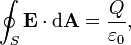# Reistance of conductive shell

Liquidxlax

## Homework Statement

Show that a conductive shell a<b has a resistance of

R= (a-1-b-1)/(4πσ)

## Homework EquationsJ = σE I = JA = σEA

R=(Va-Vb)/I

## The Attempt at a Solution

My problem is my algebra i think.

First i found the electric field

Qenc= ρV = (Qr3)/(b3-a3)

E= (4πkQenc)(4πr2) = (KQr)/(b3-a3)

solve for potential

(Va-Vb)= (kQ)/(2(a-b))

Next step is where i think i messed up

I=σEA = [(KQr)/(b3-a3)]σ(4πr2)

= (4πKQσr3)/(b3-a3)

I insert for r3 for (b3-a3)

and get 4πKQσ=I

then R= V/I

[(kq)/(2(a-b))]/(4πKQσ) = (a-1-b-1)/(8πσ)

which isn't right. thanks for the help, hope its not to messy

Mindscrape
The electric field is not quite right. You are looking between the two spheres. Since the gaussian sphere hasn't quite reached b yet, you are looking at the electric field produced by shell a. For the voltage, on the other hand, you want to integrate over the whole field from a to b.

Also that's a strange way to right the resistance. You realize it should be
$$R=\frac{1}{4\pi \epsilon_0} \left( \frac{1}{a} - \frac{1}{b} \right)$$
right?

Liquidxlax
The electric field is not quite right. You are looking between the two spheres. Since the gaussian sphere hasn't quite reached b yet, you are looking at the electric field produced by shell a. For the voltage, on the other hand, you want to integrate over the whole field from a to b.

Also that's a strange way to right the resistance. You realize it should be
$$R=\frac{1}{4\pi \epsilon_0} \left( \frac{1}{a} - \frac{1}{b} \right)$$
right?

their not concentric, its a thick shelled sphere. I wrote it the way the text book did pdf had to be in 2 files, its much cleaner

#### Attachments

• long ass equation.pdf
58.1 KB · Views: 414
• part 2.pdf
49.2 KB · Views: 172
Last edited:
Mindscrape
What do you mean they are not concentric? If they aren't concentric then good luck solving analytically. Also, what do you mean it is a thick shell? If it is a thick shell of a solid conductor then the electric field is zero and you don't have a resistance.

I think what you mean to say is, yes, they are concentric, and, there is a material between a and b with conductivity [itex]\sigma[/tex] which makes a "thick shell".

One huge problem in your first pdf (a^2-b^2)/(a^3-b^3)≠1/(a-b). The best you could do is (a+b)/(a+b)^2.

One of us has some major misconceptions about the problem. Let's see if we can clear that up first.

Liquidxlax
i pulled out my text book and this is the question exactly

7-4.4 (a) Show that the resistance of a uniform spherical shell of radii a<b and conductivity sigma, for radial current flow, is
R= (a-1-b-1)/(4(pi)sigma), (b) show that, as b-a = d --> 0 and taking A 4pia2~4pib2, R reduces to

R= (rho(l))/A where rho is inverse sigma, A is cross sectional area and l is the length for a wire.

This question is kind of cruddy for an assignment question

Mindscrape
Okay, I am pretty sure that my interpretation is correct because it gives the right answer. You have two shells, one with radius a that gives an electric field based on its charge, and another shell b that is concentric with shell a. Just give my way a try, and try to rework the problem with my previous suggestion.

To your credit, this is the type of problem that people usually see in an upper division course and never in a lower division course (granted it ought to be an easier problem for upper division).

Liquidxlax
Okay, I am pretty sure that my interpretation is correct because it gives the right answer. You have two shells, one with radius a that gives an electric field based on its charge, and another shell b that is concentric with shell a. Just give my way a try, and try to rework the problem with my previous suggestion.

To your credit, this is the type of problem that people usually see in an upper division course and never in a lower division course (granted it ought to be an easier problem for upper division).

it is a very easy problem... yet the wording is throwing me for a loop

Mindscrape
No problem, we all get tripped up now and again.

$$\mathbf{E}=\frac{1}{4 \pi \epislon_0} \frac{Q}{r^2} \matbf{\hat{r}}$$
for b>r>a

So, now voltage...

$$V = -\int \mathbf{E} \cdot dl =...$$